xThermodynamic cycleOverview

A thermodynamic cycle consists of a series of thermodynamic processes transferring heat and work, while varying pressure, temperature, and other state variables, eventually returning a system
Thermodynamic system
A thermodynamic system is a precisely defined macroscopic region of the universe, often called a physical system, that is studied using the principles of thermodynamics....

to its initial state. In the process of going through this cycle, the system may perform work on its surroundings, thereby acting as a heat engine
Heat engine
In thermodynamics, a heat engine is a system that performs the conversion of heat or thermal energy to mechanical work. It does this by bringing a working substance from a high temperature state to a lower temperature state. A heat "source" generates thermal energy that brings the working substance...

.

State quantities depend only on the thermodynamic state
Thermodynamic state
A thermodynamic state is a set of values of properties of a thermodynamic system that must be specified to reproduce the system. The individual parameters are known as state variables, state parameters or thermodynamic variables. Once a sufficient set of thermodynamic variables have been...

, and cumulative variation of such properties adds up to zero during a cycle.Unanswered QuestionsEncyclopedia
A thermodynamic cycle consists of a series of thermodynamic processes transferring heat and work, while varying pressure, temperature, and other state variables, eventually returning a system
Thermodynamic system
A thermodynamic system is a precisely defined macroscopic region of the universe, often called a physical system, that is studied using the principles of thermodynamics....

to its initial state. In the process of going through this cycle, the system may perform work on its surroundings, thereby acting as a heat engine
Heat engine
In thermodynamics, a heat engine is a system that performs the conversion of heat or thermal energy to mechanical work. It does this by bringing a working substance from a high temperature state to a lower temperature state. A heat "source" generates thermal energy that brings the working substance...

.

State quantities depend only on the thermodynamic state
Thermodynamic state
A thermodynamic state is a set of values of properties of a thermodynamic system that must be specified to reproduce the system. The individual parameters are known as state variables, state parameters or thermodynamic variables. Once a sufficient set of thermodynamic variables have been...

, and cumulative variation of such properties adds up to zero during a cycle. Process quantities (or path quantities), such as heat
Heat
In physics and thermodynamics, heat is energy transferred from one body, region, or thermodynamic system to another due to thermal contact or thermal radiation when the systems are at different temperatures. It is often described as one of the fundamental processes of energy transfer between...

and work
Work (thermodynamics)
In thermodynamics, work performed by a system is the energy transferred to another system that is measured by the external generalized mechanical constraints on the system. As such, thermodynamic work is a generalization of the concept of mechanical work in mechanics. Thermodynamic work encompasses...

are process dependent, and cumulative heat and work are non-zero. The first law of thermodynamics
First law of thermodynamics
The first law of thermodynamics is an expression of the principle of conservation of work.The law states that energy can be transformed, i.e. changed from one form to another, but cannot be created nor destroyed...

dictates that the net heat input is equal to the net work output over any cycle. The repeating nature of the process path allows for continuous operation, making the cycle an important concept in thermodynamics
Thermodynamics
Thermodynamics is a physical science that studies the effects on material bodies, and on radiation in regions of space, of transfer of heat and of work done on or by the bodies or radiation...

. Thermodynamic cycles often use quasistatic process
Quasistatic process
In thermodynamics, a quasistatic process is a thermodynamic process that happens infinitely slowly. However, it is very important of note that no real process is quasistatic...

es to model the workings of actual devices.

## Heat and work

Two primary classes of thermodynamic cycles are power cycles and heat pump cycles. Power cycles are cycles which convert some heat input into a mechanical work
Mechanical work
In physics, work is a scalar quantity that can be described as the product of a force times the distance through which it acts, and it is called the work of the force. Only the component of a force in the direction of the movement of its point of application does work...

output, while heat pump cycles transfer heat from low to high temperatures using mechanical work input. Cycles composed entirely of quasistatic processes can operate as power or heat pump cycles by controlling the process direction. On a pressure volume diagram
Pressure volume diagram
A pressure volume diagram is used to describe corresponding changes in volume and pressure in a system...

or temperature entropy diagram
Temperature entropy diagram
A temperature entropy diagram, or T-s diagram, is used in thermodynamics to visualize changes to temperature and specific entropy during a thermodynamic process or cycle. It is a useful and common tool, particularly because it helps to visualize the heat transfer during a process...

, the clockwise and counterclockwise directions indicate power and heat pump cycles, respectively.

### Relationship to workBecause the net variation in state properties during a thermodynamic cycle is zero, it forms a closed loop on a PV diagram. A PV diagram's Y axis shows pressure (P) and X axis shows volume (V). The area enclosed by the loop is the work (W) done by the process: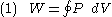This work is equal to the balance of heat (Q) transferred into the system: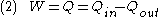Equation (2) makes a cyclic process similar to an isothermal process
Isothermal process
An isothermal process is a change of a system, in which the temperature remains constant: ΔT = 0. This typically occurs when a system is in contact with an outside thermal reservoir , and the change occurs slowly enough to allow the system to continually adjust to the temperature of the reservoir...

: even though the internal energy changes during the course of the cyclic process, when the cyclic process finishes the system's energy is the same as the energy it had when the process began.

If the cyclic process moves clockwise around the loop, then W will be positive, and it represents a heat engine
Heat engine
In thermodynamics, a heat engine is a system that performs the conversion of heat or thermal energy to mechanical work. It does this by bringing a working substance from a high temperature state to a lower temperature state. A heat "source" generates thermal energy that brings the working substance...

. If it moves counterclockwise, then W will be negative, and it represents a heat pump
Heat pump
A heat pump is a machine or device that effectively "moves" thermal energy from one location called the "source," which is at a lower temperature, to another location called the "sink" or "heat sink", which is at a higher temperature. An air conditioner is a particular type of heat pump, but the...

.

### Power cycles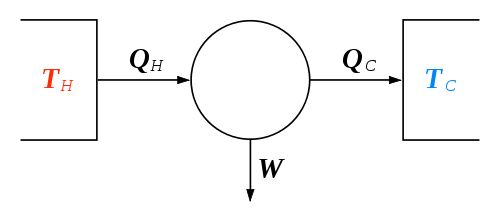Thermodynamic power cycles are the basis for the operation of heat engines, which supply most of the world's electric power
Electric power
Electric power is the rate at which electric energy is transferred by an electric circuit. The SI unit of power is the watt.-Circuits:Electric power, like mechanical power, is represented by the letter P in electrical equations...

and run the vast majority of motor vehicles. Power cycles can be divided according to the type of heat engine they seek to model. The most common cycles that model internal combustion engines are the Otto cycle
Otto cycle
An Otto cycle is an idealized thermodynamic cycle which describes the functioning of a typical reciprocating piston engine, the thermodynamic cycle most commonly found in automobile engines....

, which models gasoline engines and the Diesel cycle
Diesel cycle
The Diesel cycle is the thermodynamic cycle which approximates the pressure and volume of the combustion chamber of the Diesel engine, invented by Rudolph Diesel in 1897. It is assumed to have constant pressure during the first part of the "combustion" phase...

, which models diesel engines. Cycles that model external combustion engines include the Brayton cycle
Brayton cycle
The Brayton cycle is a thermodynamic cycle that describes the workings of the gas turbine engine, basis of the airbreathing jet engine and others. It is named after George Brayton , the American engineer who developed it, although it was originally proposed and patented by Englishman John Barber...

, which models gas turbines, and the Rankine cycle
Rankine cycle
The Rankine cycle is a cycle that converts heat into work. The heat is supplied externally to a closed loop, which usually uses water. This cycle generates about 90% of all electric power used throughout the world, including virtually all solar thermal, biomass, coal and nuclear power plants. It is...

, which models steam turbines.For example the pressure-volume mechanical work
Mechanical work
In physics, work is a scalar quantity that can be described as the product of a force times the distance through which it acts, and it is called the work of the force. Only the component of a force in the direction of the movement of its point of application does work...

done in the heat engine cycle, consisting of 4 thermodynamic processes, is: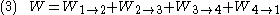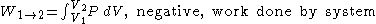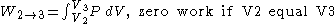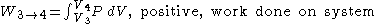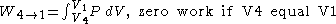If no volume change happens in process 4->1 and 2->3, equation (3) simplifies to: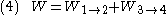### Heat pump cycles

Thermodynamic heat pump cycles are the model
Mathematical model
A mathematical model is a description of a system using mathematical concepts and language. The process of developing a mathematical model is termed mathematical modeling. Mathematical models are used not only in the natural sciences and engineering disciplines A mathematical model is a...

s for heat pumps and refrigerators. The difference between the two is that heat pumps are intended to keep a place warm while refrigerators are designed to cool it. The most common refrigeration cycle is the vapor compression cycle, which models systems using refrigerants that change phase. The absorption refrigeration cycle is an alternative that absorbs the refrigerant in a liquid solution rather than evaporating it. Gas refrigeration cycles include the reversed Brayton cycle and the Hampson-Linde cycle
Hampson-Linde cycle
The Hampson–Linde cycle is based on the Joule-Thomson effectand is used in the liquefaction of gases. W. Hampson and Carl von Linde independently filed for patent of the cycle in 1895.-External links:*...

. Regeneration in gas refrigeration allows for the liquefaction of gases
Liquefaction of gases
Liquefaction of gases includes a number of phases used to convert a gas into a liquid state. The processes are used for scientific, industrial and commercial purposes. Many gases can be put into a liquid state at normal atmospheric pressure by simple cooling; a few, such as carbon dioxide, require...

.

## Modelling real systems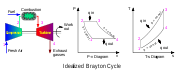Thermodynamic cycles may be used to model real devices and systems, typically by making a series of assumptions. simplifying assumptions are often necessary to reduce the problem to a more manageable form. For example, as shown in the figure, devices such a gas turbine
Gas turbine
A gas turbine, also called a combustion turbine, is a type of internal combustion engine. It has an upstream rotating compressor coupled to a downstream turbine, and a combustion chamber in-between....

or jet engine
Jet engine
A jet engine is a reaction engine that discharges a fast moving jet to generate thrust by jet propulsion and in accordance with Newton's laws of motion. This broad definition of jet engines includes turbojets, turbofans, rockets, ramjets, pulse jets...

can be modelled as a Brayton cycle
Brayton cycle
The Brayton cycle is a thermodynamic cycle that describes the workings of the gas turbine engine, basis of the airbreathing jet engine and others. It is named after George Brayton , the American engineer who developed it, although it was originally proposed and patented by Englishman John Barber...

. The actual device is made up of a series of stages, each of which is itself modelled as an idealized thermodynamic process. Although each stage which acts on the working fluid is a complex real device, they may be modelled as idealized processes which approximate their real behavior. A further assumption is that the exhaust gases would be passed back through the inlet with a corresponding loss of heat, thus completing the idealized cycle.

The difference between an idealized cycle and actual performance may be significant. For example, the following images illustrate the differences in work output predicted by an ideal Stirling cycle
Stirling cycle
The Stirling cycle is a thermodynamic cycle that describes the general class of Stirling devices. This includes the original Stirling engine that was invented, developed and patented in 1816 by Reverend Dr...

and the actual performance of a Stirling engine:
 Ideal Stirling cycle Actual performance Actual and ideal overlaid, showing difference in work output

As work output is represented by the interior of the cycle, there is a significant difference between the predicted work output of the ideal cycle and the actual work output shown by a real engine. It may also be observed that the real individual processes diverge from their idealized counterparts; e.g., isochoric expansion (process 1-2) occurs with some actual volume change.

## Well-known thermodynamic cycles

In practice, simple idealized thermodynamic cycles are usually made out of four thermodynamic processes. Any thermodynamic processes may be used. However, when idealized cycles are modeled, often processes where one state variable is kept constant are used, such as an isothermal process
Isothermal process
An isothermal process is a change of a system, in which the temperature remains constant: ΔT = 0. This typically occurs when a system is in contact with an outside thermal reservoir , and the change occurs slowly enough to allow the system to continually adjust to the temperature of the reservoir...

(constant temperature), isobaric process
Isobaric process
An isobaric process is a thermodynamic process in which the pressure stays constant. The term derives from the Greek isos, , and barus,...

(constant pressure), isochoric process
Isochoric process
An isochoric process, also called a constant-volume process, an isovolumetric process, or an isometric process, is a thermodynamic process during which the volume of the closed system undergoing such a process remains constant...

(constant volume), isentropic process
Isentropic process
In thermodynamics, an isentropic process or isoentropic process is one in which for purposes of engineering analysis and calculation, one may assume that the process takes place from initiation to completion without an increase or decrease in the entropy of the system, i.e., the entropy of the...

(constant entropy), or an isenthalpic process
Isenthalpic process
An isenthalpic process or isoenthalpic process is a process that proceeds without any change in enthalpy, H; or specific enthalpy, h....

(constant enthalpy). Often adiabatic process
Adiabatic process
In thermodynamics, an adiabatic process or an isocaloric process is a thermodynamic process in which the net heat transfer to or from the working fluid is zero. Such a process can occur if the container of the system has thermally-insulated walls or the process happens in an extremely short time,...

es are also used, where no heat is exchanged.

Some example thermodynamic cycles and their constituent processes are as follows:

### Ideal cycle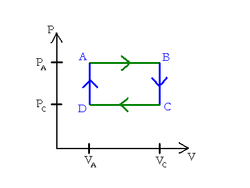An ideal cycle is constructed out of:
1. TOP and BOTTOM of the loop: a pair of parallel isobaric processes
2. LEFT and RIGHT of the loop: a pair of parallel isochoric processes

### Carnot cycle

The Carnot cycle
Carnot cycle
The Carnot cycle is a theoretical thermodynamic cycle proposed by Nicolas Léonard Sadi Carnot in 1824 and expanded by Benoit Paul Émile Clapeyron in the 1830s and 40s. It can be shown that it is the most efficient cycle for converting a given amount of thermal energy into work, or conversely,...

is a cycle composed of the totally reversible processes of isentropic compression and expansion and isothermal heat addition and rejection. The thermal efficiency
Thermal efficiency
In thermodynamics, the thermal efficiency is a dimensionless performance measure of a device that uses thermal energy, such as an internal combustion engine, a boiler, a furnace, or a refrigerator for example.-Overview:...

of a Carnot cycle depends only on the absolute temperatures of the two reservoirs in which heat transfer takes place, and for a power cycle is: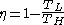where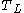is the lowest cycle temperature and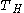the highest. For Carnot power cycles the coefficient of performance for a heat pump
Heat pump
A heat pump is a machine or device that effectively "moves" thermal energy from one location called the "source," which is at a lower temperature, to another location called the "sink" or "heat sink", which is at a higher temperature. An air conditioner is a particular type of heat pump, but the...

is: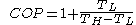and for a refrigerator
Refrigerator
A refrigerator is a common household appliance that consists of a thermally insulated compartment and a heat pump that transfers heat from the inside of the fridge to its external environment so that the inside of the fridge is cooled to a temperature below the ambient temperature of the room...

the coefficient of performance is: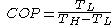The second law of thermodynamics limits the efficiency and COP for all cyclic devices to levels at or below the Carnot efficiency. The Stirling cycle
Stirling cycle
The Stirling cycle is a thermodynamic cycle that describes the general class of Stirling devices. This includes the original Stirling engine that was invented, developed and patented in 1816 by Reverend Dr...

and Ericsson cycle
Ericsson cycle
The Ericsson cycle is named after inventor John Ericsson, who designed and built many unique heat engines based on various thermodynamic cycles. He is credited with inventing two unique heat engine cycles and developing practical engines based on these cycles...

are two other reversible cycles that use regeneration to obtain isothermal heat transfer.

### Stirling cycle

A Stirling cycle is like an Otto cycle, except that the adiabats are replaced by isotherms. It is also the same as an Ericsson cycle with the isobaric processes substituted for constant volume processes.
1. TOP and BOTTOM of the loop: a pair of quasi-parallel isothermal processes
2. LEFT and RIGHT sides of the loop: a pair of parallel isochoric processes

Heat flows into the loop through the top isotherm and the left isochore, and some of this heat flows back out through the bottom isotherm and the right isochore, but most of the heat flow is through the pair of isotherms. This makes sense since all the work done by the cycle is done by the pair of isothermal processes, which are described by Q=W. This suggests that all the net heat comes in through the top isotherm. In fact, all of the heat which comes in through the left isochore comes out through the right isochore: since the top isotherm is all at the same warmer temperatureand the bottom isotherm is all at the same cooler temperature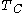, and since change in energy for an isochore is proportional to change in temperature, then all of the heat coming in through the left isochore is cancelled out exactly by the heat going out the right isochore.

## State functions and entropy

If Z is a state function
State function
In thermodynamics, a state function, function of state, state quantity, or state variable is a property of a system that depends only on the current state of the system, not on the way in which the system acquired that state . A state function describes the equilibrium state of a system...

then the balance of Z remains unchanged during a cyclic process: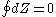.

Entropy is a state function and is defined as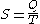so that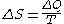,
then it is clear that for any cyclic process,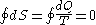meaning that the net entropy change over a cycle is 0.

## Further reading

• Halliday, Resnick & Walker. Fundamentals of Physics, 5th edition. John Wiley & Sons, 1997. Chapter 21, Entropy and the Second Law of Thermodynamics.
The source of this article is wikipedia, the free encyclopedia.  The text of this article is licensed under the GFDL.